﻿ 海洋平台用EH460钢热变形行为研究 Study on Hot Deformation Behavior of EH460 Steel for Offshore Platform

Material Sciences
Vol. 12  No. 04 ( 2022 ), Article ID: 51011 , 10 pages
10.12677/MS.2022.124043

1湖南华菱湘潭钢铁有限公司技术质量部，湖南 湘潭

2北京科技大学钢铁共性技术协同创新中心，北京EH460钢，热变形行为，真应力–真应变曲线，本构方程，热加工图Study on Hot Deformation Behavior of EH460 Steel for Offshore Platform

Chende Tuo1, Deng Luo1, Daheng Xiao1, Yongwei Zhang1, Huibin Wu2

1Department of Technical Quality, Xiangtan Iron & Steel Co., Ltd. of Hunan Valin, Xiangtan Hunan

2Collaborative Innovation Center of Steel Technology, University of Science and Technology Beijing, Beijing

Received: Mar. 23rd, 2022; accepted: Apr. 22nd, 2022; published: Apr. 29th, 2022ABSTRACT

The hot compression test of EH460 steel was carried out on Gleeble-1500 thermal simulator to investigate the hot deformation behavior of the steel. The deformation temperature was 900˚C~ 1150˚C and the deformation rate was 0.01~10 s−1. The results show that the main deformation mechanisms of EH460 steel are work hardening, dynamic recovery and dynamic recrystallization from the true stress-true strain curves. The flow stress decreases with the increase of deformation temperature and increases with the increase of strain rate. The flow stress under different deformation conditions is calculated by constructing the constitutive equation. The correlation coefficient between the predicted value and the experimental value is 0.994 and the average relative error is 4.498%. The correlation is good. By analyzing the hot processing map, it is concluded that the parameters suitable for hot working of EH460 steel are mainly 950˚C~1080˚C, 0.1~10 s−1, 1040˚C~1080˚C, 0.01~0.1 s−1 and 1130˚C~1150˚C, 0.01~0.1 s−1.

Keywords:EH460 Steel, Hot Deformation Behavior, True Stress-True Strain Curves, Constitutive Equation, Hot Processing Map1. 引言

2. 材料与方法Table 1. Chemical composition of EH460 steel (mass fraction, %)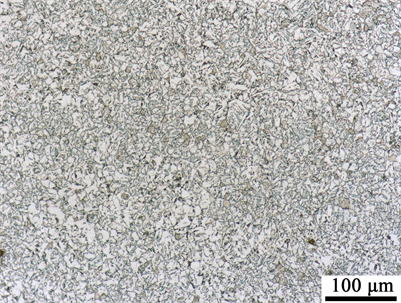Figure 1. Original microstructure of EH460 steel

3. 结果与讨论

3.1. 真应力–真应变曲线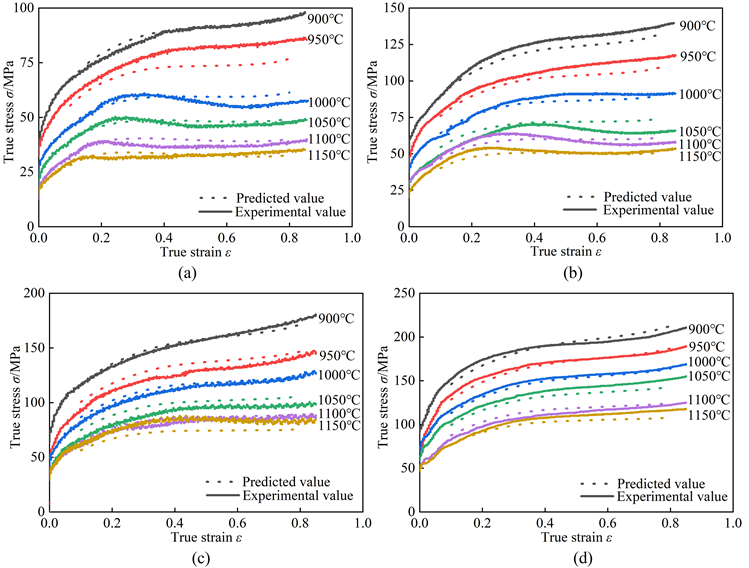Figure 2. True stress-true strain curves and predicted value of EH460 steel (a) 0.01 s−1; (b) 0.1 s−1; (c) 1 s−1; (d) 10 s−1

3.2. 加工硬化率

3.3. 构建本构方程

$Z=\stackrel{˙}{\epsilon }\mathrm{exp}\left(\frac{Q}{RT}\right)=\left\{\begin{array}{l}{A}_{1}{\sigma }^{{n}_{1}},\text{}\alpha \sigma \le 0.8\hfill \\ {A}_{2}\mathrm{exp}\left(\beta \sigma \right),\text{}\alpha \sigma \ge 1.2\hfill \\ A{\left[\mathrm{sinh}\left(\alpha \sigma \right)\right]}^{n},\text{forall}\sigma \hfill \end{array}$ (1)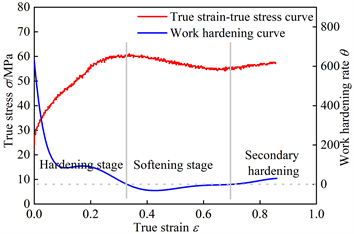Figure 3. True stress-true strain and work hardening rate; 1000˚C, 0.01 s−1

$\mathrm{ln}Z=\mathrm{ln}\stackrel{˙}{\epsilon }+\frac{Q}{RT}=\left\{\begin{array}{l}\mathrm{ln}{A}_{1}+{n}_{1}\mathrm{ln}\sigma ,\text{}\alpha \sigma \le 0.8\hfill \\ \mathrm{ln}{A}_{2}+\beta \sigma ,\text{}\alpha \sigma \ge 1.2\hfill \\ \mathrm{ln}A+n\mathrm{ln}\left[\mathrm{sinh}\left(\alpha \sigma \right)\right],\text{forall}\sigma \hfill \end{array}$ (2)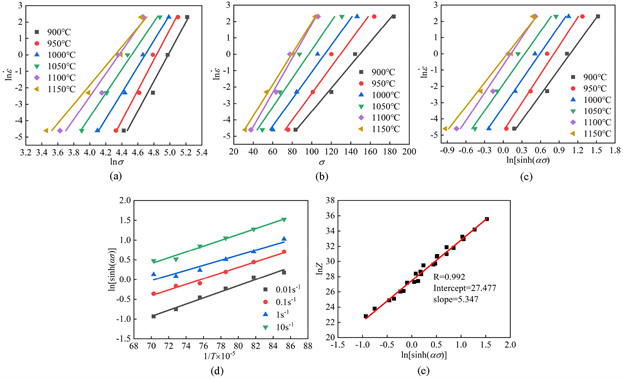Figure 4. Linear fitting diagram of thermal deformation coefficient (a) n1; (b) β; (c) n; (d) Q; (e) lnA

ε = 0.3时，n1值取1150℃时线性拟合直线 $\mathrm{ln}\stackrel{˙}{\epsilon }-\mathrm{ln}\sigma$ 的斜率，β值取900℃时线性拟合直线 $\mathrm{ln}\stackrel{˙}{\epsilon }-\sigma$ 的斜率；nQA分别取 $\mathrm{ln}\stackrel{˙}{\epsilon }-\mathrm{ln}\left[\mathrm{sinh}\left(\alpha \sigma \right)\right]$$\mathrm{ln}\left[\mathrm{sinh}\left(\alpha \sigma \right)\right]-1/T$$\mathrm{ln}Z-\mathrm{ln}\left[\mathrm{sinh}\left(\alpha \sigma \right)\right]$ 中所有线性拟合直线斜率的平均值。由各拟合直线的斜率最终可得αnQ、lnA分别为α = β/n1 = 0.0121 MPa−1n = 5.435、Q = 324.284 kJ∙mol−1、lnA = 27.477 s−1图4(e)中拟合直线的斜率即为n，其值为5.347，与图4(c)所得n的相对差为1.6%，在合理误差范围内。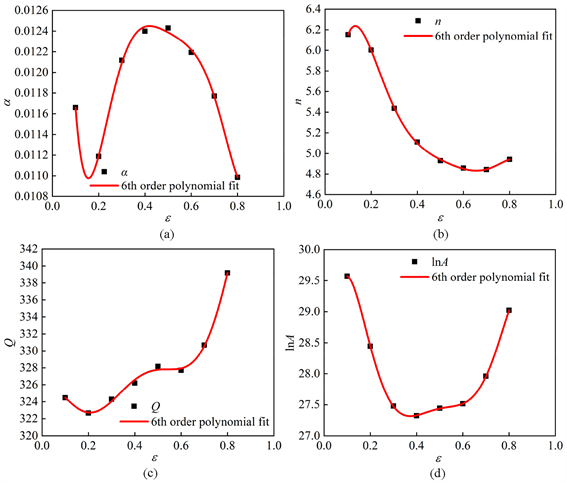Figure 5. The relation curves between thermal deformation coefficient and strain (a) α; (b) n; (c) Q; (d) lnATable 2. Thermal deformation coefficient under different strain conditions

$\left\{\begin{array}{l}\alpha ={B}_{0}+{B}_{1}\epsilon +{B}_{2}{\epsilon }^{2}+{B}_{3}{\epsilon }^{3}+{B}_{4}{\epsilon }^{4}+{B}_{5}{\epsilon }^{5}+{B}_{6}{\epsilon }^{6}\hfill \\ n={C}_{0}+{C}_{1}\epsilon +{C}_{2}{\epsilon }^{2}+{C}_{3}{\epsilon }^{3}+{C}_{4}{\epsilon }^{4}+{C}_{5}{\epsilon }^{5}+{C}_{6}{\epsilon }^{6}\hfill \\ Q={D}_{0}+{D}_{1}\epsilon +{D}_{2}{\epsilon }^{2}+{D}_{3}{\epsilon }^{3}+{D}_{4}{\epsilon }^{4}+{D}_{5}{\epsilon }^{5}+{D}_{6}{\epsilon }^{6}\hfill \\ \mathrm{ln}A={E}_{0}+{E}_{1}\epsilon +{E}_{2}{\epsilon }^{2}+{E}_{3}{\epsilon }^{3}+{E}_{4}{\epsilon }^{4}+{E}_{5}{\epsilon }^{5}+{E}_{6}{\epsilon }^{6}\hfill \end{array}$ (3)

$\sigma =\frac{1}{\alpha \left(\epsilon \right)}\mathrm{ln}\left\{{\left(\frac{Z\left(\epsilon \right)}{A\left(\epsilon \right)}\right)}^{\frac{1}{n\left(\epsilon \right)}}+{\left[{\left(\frac{Z\left(\epsilon \right)}{A\left(\epsilon \right)}\right)}^{\frac{2}{n\left(\epsilon \right)}}+1\right]}^{\frac{1}{2}}\right\}$ (4)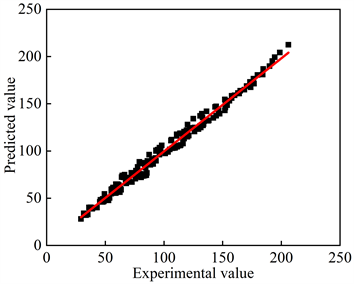Figure 6. Correlation between experimental and predicted stresses

$R=\frac{{\sum }_{i=1}^{N}\left({E}_{i}-\stackrel{¯}{E}\right)\left({P}_{i}-\stackrel{¯}{P}\right)}{\sqrt{{\sum }_{i=1}^{N}{\left({E}_{i}-\stackrel{¯}{E}\right)}^{2}{\sum }_{i=1}^{N}{\left({P}_{i}-\stackrel{¯}{P}\right)}^{2}}}$ (5)

$AARE\left(%\right)=\frac{1}{N}{\sum }_{i=1}^{N}|\frac{{E}_{i}-{P}_{i}}{{E}_{i}}|×100%$ (6)

3.4. 热加工图

$\sigma =K{\stackrel{˙}{\epsilon }}^{m}$ (7)

$\mathrm{ln}\sigma ={a}_{1}+{a}_{2}\left(\mathrm{ln}\stackrel{˙}{\epsilon }\right)+{a}_{3}{\left(\mathrm{ln}\stackrel{˙}{\epsilon }\right)}^{2}+{a}_{4}{\left(\mathrm{ln}\stackrel{˙}{\epsilon }\right)}^{3}$ (8)

$m={\frac{\partial \left(\mathrm{ln}\sigma \right)}{\partial \left(\mathrm{ln}\stackrel{˙}{\epsilon }\right)}|}_{T,\epsilon }={a}_{2}+2{a}_{3}\left(\mathrm{ln}\stackrel{˙}{\epsilon }\right)+3{a}_{4}{\left(\mathrm{ln}\stackrel{˙}{\epsilon }\right)}^{2}$ (9)

m还可表示为耗散协量和能量耗散量的比值，故m的范围为0~1，一般希望m接近1较好，说明材料的塑性好。要想准确判断材料的变形机制还需参考功率耗散因子η和失稳判据ξ。功率耗散因子η可反映微观组织转变的机理，一般认为η较大时更易发生动态回复和动态再结晶等，热加工性能更好 。η可用变形速率敏感指数m表示为式(10)：

$\eta =\frac{2m}{m+1}$ (10)

$\xi =\frac{\partial \mathrm{ln}\left(\frac{m}{m+1}\right)}{\partial \mathrm{ln}\stackrel{˙}{\epsilon }}+m<0$ (11)

3.5. 微观组织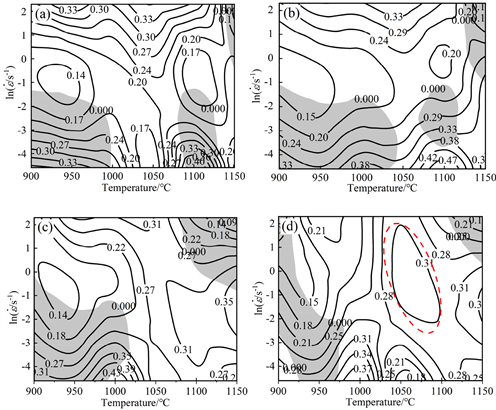Figure 7. Hot processing map of EH460 steel under different strain (a) 0.2; (b) 0.4; (c) 0.6; (d) 0.8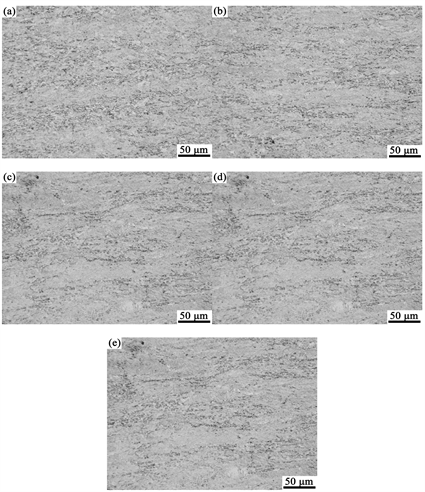Figure 8. Microstructure of the sample under different deformation conditions. (a) 1050˚C, 0.01 s−1; (b) 1050˚C, 0.1 s−1; (c) 1050˚C, 1 s−1; (d) 1100˚C, 0.01 s−1; (e) 1150˚C, 0.01 s−1

4. 结论

1) EH460钢的热变形行为主要受加工硬化、动态回复和动态再结晶控制，真应力–真应变曲线也表现出不同的变形机制，且流变应力随变形温度的增大而减小，随变形速率的增大而增大。

2) 通过建立EH460钢的本构方程，计算出在不同变形条件下的预测值，将其与试验值进行对比，R值为0.994，AARE值为4.498%，相关性较好。

3) 基于DMM模型分析了EH460钢在不同应变量下的热加工图，并通过观察不同变形条件下的显微组织进行验证，适合热加工的工艺区间主要为950℃~1080℃、0.1~10 s−1、1040℃~1080℃、0.01~0.1 s−1和1130℃~1150℃、0.01~0.1 s−1

Study on Hot Deformation Behavior of EH460 Steel for Offshore Platform[J]. 材料科学, 2022, 12(04): 409-418. https://doi.org/10.12677/MS.2022.124043

1. 1. 杨春卫, 宋增强. 80 mm调质海工钢EH550的生产开发与应用[J]. 中国金属通报, 2021(3): 151-152.

2. 2. 李海军, 李天祥, 李睿昊, 等. 直接轧制工艺对中厚板组织与性能的影响[J]. 钢铁, 2021, 56(4): 64-69.

3. 3. 刘朋. 低钢温轧制工艺在中厚板生产中的应用[J]. 宽厚板, 2020, 26(4): 27-30.

4. 4. 张兵, 刘鹏茹, 陈韩锋, 等. 铸态GH2132合金热变形行为和热加工图研究[J]. 中国有色金属学报, 2022, 32(2): 466-475.

5. 5. 孔晓寒, 陈慧琴, 刘建生, 等. 铸态Q345E钢的本构方程及动态再结晶行为[J]. 锻压技术, 2020, 45(11): 199-204.

6. 6. 王顺, 李玉贵, 宋耀辉, 等. 2205/Q345热压缩复合热变形行为的研究[J]. 重型机械, 2020(5): 65-69.

7. 7. Xu, L.X., Wu, H.B. and Wang, X.T. (2018) Influence of Microstructural Evolution on the Hot Deformation Behavior of an Fe-Mn-Al Duplex Lightweight Steel. Acta Metallurgica Sinica, 31, 389-400. https://doi.org/10.1007/s40195-017-0655-7

8. 8. 许立雄. Fe-Mn-Al系轻质低温钢的组织调控及强韧化机理研究[D]: [博士学位论文]. 北京: 北京科技大学, 2019.

9. 9. 储滔, 沈慧, 斯庭智. 30CrNi3MoV钢的热变形行为及热加工图[J]. 金属热处理, 2020, 45(10): 24-30.

10. 10. Zhang, L.B., Liu, G.M., Han, T.Z., et al. (2021) Microstructural Evolution and Strain-Compensated Constitutive Equation for As-Cast Mg-3Sn-1Mn-1La Alloy during Isothermal Compression. Rare Metal Materials and Engneering, 50, 835-847.

11. 11. Tan, G., Li, H.z., Wang, Y., et al. (2020) Hot Working Characteristics of HEXed PM Nickel-Based Superalloy during Hot Compression. Transactions of Nonferrous Metals Society of China, 30, 2709-2723. https://doi.org/10.1016/S1003-6326(20)65414-0

12. 12. Xi, S.P., Gao, X.L., Liu, W., et al. (2022) Hot Deformation Behavior and Processing Map of Low-Alloy Offshore Steel. Journal of Iron and Steel Research International, 29, 474-483. https://doi.org/10.1007/s42243-021-00603-4

13. 13. Poliak, E.I. and Jonas, J.J. (1996) A One-Parameter Approach to Determining the Critical Conditions for the Initiation of Dynamic Recrystallization. Acta Materialia, 44, 127-136. https://doi.org/10.1016/1359-6454(95)00146-7

14. 14. Chen, Y.Z., Pang, Y.H., Wang, J.G., et al. (2020) Hot Deformation Behavior and Hot Processing Map of GH2907 Superalloy. Rare Metal Materials and Engineering, 49, 2956-2965.